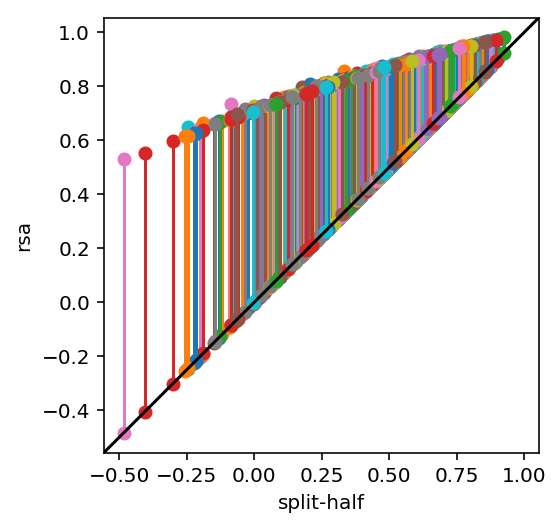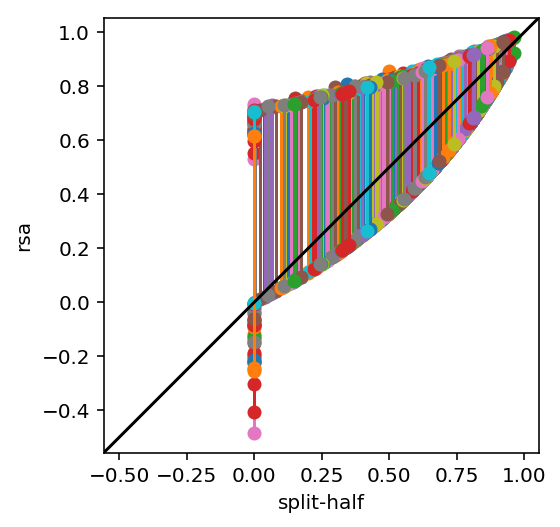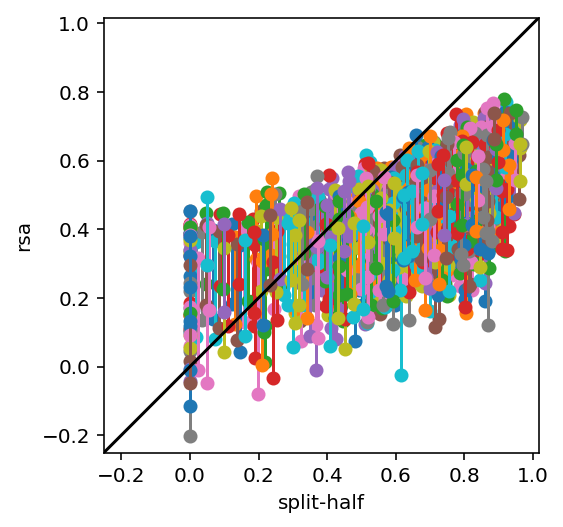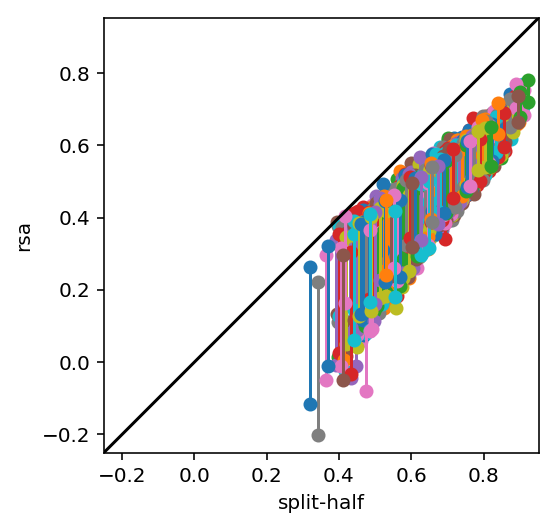Last year I reviewed a nice paper on noise ceiling methods (now published - Lage-Castellanos et al., 2019). I wrote the below notebook to help me understand the authors' methods, but I realised it might be useful for other people, so here you are. An interactive version of this notebook is available on collaboratory.

It is increasingly popular in computational neuroimaging to report model prediction performance with regard to an estimated noise ceiling. The logic underlying this approach is that stimulus responses are fixed and differ over repetitions only in noise. Under this assumption, one can estimate how close the evaluated model's performance gets to the maximal possible performance given noise levels in the data. This is especially useful in noninvasive neuroimaging where contrast-to-noise ratios often are low, and consequently raw prediction performances (by which I mean pearson r for the purposes of this notebook) are expected to be bounded by this such that even the true model will have a far from perfect prediction performance.

The basic idea with noise ceilings is to estimate how much stimulus-related variance you have in the data, that is, how much variance could you explain if you had the true, data-generating model? The assumption underlying this is that stimulus responses are fixed, and differ only in noise. So we can see why noise ceilings are popular in sensory neuroscience but not in, say, learning and memory, where change in representation over repetitions is the variable of interest rather than noise.

Sometimes people even adjust their reported effects for the noise ceiling. So for instance, if you have a correlation between data and model of 0.3 but your noise ceiling estimate is 0.4, you obtain a 'corrected' correlation of $0.3 / 0.4 = 0.75$, which sounds much better, but obscures what your noise ceiling actually was. Which is relevant for evaluating the quality of your data. So please don't do this.

## 'RSA' noise ceiling¶

In the representational similarity analysis (RSA) literature, noise ceilings are usually estimated at the group-average level. We estimate the distance matrix for each subject (or session, for a single subject analysis). We perform leave-one-out cross-validation over these distance matrices. For each split, we compare the left-out distance matrix to the average over the remaining distance matrices to get the lower noise ceiling, and to the average of all distance matrices (including the left-out one!) as the upper noise ceiling (Nili et al., 2014). The average over all splits is our final estimate for the noise ceiling, which is a range from low to high rather than a point estimate.

It can be proved that if we had the true, data-generating model, the average correlation between its distance matrix and the distance matrix for our sample would fall in the noise ceiling range. Intuitively, the lower noise ceiling is an underestimate of the true noise ceiling, because we couldn't use all the data in the training split, and the upper noise ceiling is an overestimate, because we are using the same data in train and test, which introduces a slight circularity. We can imagine that if we are estimating an average over many distance matrices, the noise ceiling range is going to get tighter, because it's going to make less and less difference whether that one distance matrix was taken out (for the lower) or left in (for the upper).

## Noise ceilings in encoding models¶

In the encoding model literature, noise ceilings are usually estimated for a single point estimate (for instance, one correlation coefficient, rather than its average over subjects as in the RSA noise ceiling case). Methods for doing this fall into two categories: parametric methods, which are often estimated with Monte Carlo simulations (the Lage-Castellanos et al paper proposes an elegant analytical form instead), and non-parametric split-half methods that use the Spearman-Brown correction (SB). I like the latter method because it is intuitive, and makes minimal assumptions (perhaps at the cost of greater variability compared to the parametric approach above).

The Spearman-Brown formula approximates what correlation we would expect to observe if we had the same effect, but over a larger (or smaller) sample. The formula is quite simple:

$$s = \frac{(k * r)}{(1 + (k - 1) * r)}$$

Where $r$ is the observed correlation, and $k$ is the scale factor on the sample size. So to get a noise ceiling estimate, we split the test data in half and correlate the halves (for instance, over conditions or unique elements in a distance matrix). Then we plug this into the SB formula for $k=2$ to obtain an estimate of how much the data would be expected to correlate with itself if we had twice as much data, that is, another replicate of the full test split. Which is then a sensible quantity to compare our model predictions to (since they were also estimated over the full test split). Easy!

# What are we doing today?¶

We will simulate some data, and run it through SB and RSA noise ceilings. We will understand that these estimates will be different, because they are estimating different quantities. Along the way we will see how to do some basic cross-validation and RSA using numpy only.

In :
import numpy as np
import matplotlib.pyplot as plt
plt.ion()
%config InlineBackend.figure_format = 'retina'
import matplotlib
matplotlib.rcParams['figure.figsize'] = [10, 10]
from IPython.core.debugger import set_trace

In :
# parameters
nrun = 2
ncon = 12
signallev = 1
nsim = 1000

In :
# functions
def spearmanbrown(rho, k):
"""adjust the correlation coefficient r for test length by scaling by k, which
defines the full length (e.g., if the full length is twice of what r was
calculated for, k=2)."""
# SB is undefined if r<0
thisr = rho * np.array(rho > 0., dtype='float')
thisr = (k * thisr) / (1 + (k - 1) * thisr)
return thisr

def splitter(b):
"""split the data NFold, leaving out one element of b (first dim) each time.
Returns the mean over the training split (axis=1) and the test split."""
nsplit = b.shape
allind = np.arange(nsplit)
for testind in allind:
trainind = np.setdiff1d(allind, testind)
yield np.mean(b[:,trainind], axis=1), b[:, testind]

def r(b1, b2):
"""correlation coefficient without obnoxious matrix return"""
return np.corrcoef(x=b1, y=b2)

def rz(b1, b2):
"""fisher Z-transformed correlation between vectors b1 and b2."""
return np.arctanh(r(b1, b2))

def rsaceiling(b):
"""calculate RSA noise ceiling by running KFold splits on b."""
# lower ceiling is just KFold CV
lower = [rz(train, test) for train, test in splitter(b)]
# upper is similar, but use the mean across the data instead of train
upper = [rz(np.mean(b, axis=1), test) for _, test in splitter(b)]
return np.tanh(np.mean(lower)), np.tanh(np.mean(upper))

def sbceiling(b):
"""calculate SB noise ceiling by running odd-even splits (plus averaging) on b."""
# odd-even split
btrain = np.mean(b[:,0::2], axis=1)
btest = np.mean(b[:,1::2], axis=1)
thisr = r(btrain, btest)
return spearmanbrown(thisr, 2), thisr

def sbceiling_comparable(b):
"""calculate SB noise ceiling without averaging - just get all the unique n x n
correlations, correct those and get the estimate."""
# let's just correlate everything without averaging
rmat = np.corrcoef(b.T)
# don't call it squareform
rvec = rmat[np.triu_indices_from(rmat, 1)]
# nb now the correction is stronger because we map from 1 run to all the runs
correction = b.shape
rvec_sb = spearmanbrown(rvec, correction)
rvec_sb[rvec_sb<0] = np.nan
# Z-transform, average, and back to original units
return np.tanh(np.nanmean(np.arctanh(rvec_sb)))

def simulation():
"""cook data and run one iteration of the simulation."""
signal = np.random.standard_normal((ncon,1)) * signallev
thisb = signal + np.random.standard_normal((ncon, nrun))
rsalow, rsahigh = rsaceiling(thisb)
sb, rawr = sbceiling(thisb)
sbcomp = sbceiling_comparable(thisb)
return (rsalow,rsahigh), (sb, rawr), sbcomp, thisb

def plotresults(res_rsa, res_rb):
"""scatter of noise ceilings over iterations, draw unity line."""
fig, ax = plt.subplots(1, 1)
ph = ax.plot(np.repeat(res_rb[:,None], 2, axis=1).T, res_rsa.T, '-o')
axlim = ax.axis()
axmin = np.min(axlim)
axmax = np.max(axlim)
ax.set_xlim(axmin, axmax)
ax.set_ylim(axmin, axmax)
ax.set_aspect('equal')
ax.plot([axmin, axmax], [axmin, axmax], '-k')
ax.set_xlabel('split-half')
ax.set_ylabel('rsa')
return


We start by running some very simple simulations. We have responses to ncon conditions from each of nrun sessions. There is a consistent signal over the sessions, which is corrupted by additive Gaussian noise.

In :
# run initial simulation - how do the noise ceiling estimates compare for the
# simplest case (only 2 runs to split over)?
res_rsa, res_rb, _, simb = zip(*[simulation() for sim in range(nsim)])
res_rsa = np.array(res_rsa)
res_rb = np.array(res_rb)
# unpack raw and SB-corrected correlation coefficients
res_rb, res_rb_raw = res_rb[:,0], res_rb[:,1]


Now we visualise the RSA against split-half correlation over iterations of the simulation. Each line is connects the upper and lower RSA noise ceiling for one iteration.

In :
plotresults(res_rsa, res_rb_raw)Notice thate the split-half estimate (before SB) is exactly the lower-bound rsa noise ceiling (since there's only 2 runs to split, so in both cases we're just correlating them).

And after applying the SB correction to the split-half correlation we get:

In :
plotresults(res_rsa, res_rb)Notice the nonlinearity. The weirdness at the left end of the plot happens because the SB correction is undefined for r<0.

You might think from this that the SB ceiling is always going to be in the RSA noise ceiling range. But that's not true when you have more runs to split over:

In :
nrun = 10
# lower CNR to avoid saturation
signallev = .5

res_rsa10, res_rb10, res_rb_comp10, simb = zip(*[simulation() for sim in range(nsim)])
res_rsa10 = np.array(res_rsa10)
res_rb10 = np.array(res_rb10)
res_rb10, res_rb10_raw = res_rb10[:,0], res_rb10[:,1]
res_rb_comp10 = np.array(res_rb_comp10)

In :
plotresults(res_rsa10, res_rb10)Woah, what happened? Well, there's two things going on here that makes the estimates only weakly associated.

1. the RSA ceiling is based on leaving 1/10 out, while the SB is a split-half (5/5)
2. the RSA ceiling is an average over all those splits, while the SB is a one-shot

So here's an alternative way to calculate the SB ceiling. Let's just correlate ALL the individual 10 runs with each other, correct the single run estimates up to the full size (ie k=10!). So this deals with issue 1 above. (compare sbceiling and sbceiling_comparable)

In :
plotresults(res_rsa10, res_rb_comp10)And we can see this restores some order. There is now a decent linear relationship between the two noise ceilings over iterations, as you would expect. But why is the RSA noise ceiling consistently lower? The answer is issue 2 above. The SB noise ceiling is an estimate of how strongly the true model would correlate with the entire 10-run dataset, if we ran the correlation once. The RSA noise ceiling is an estimate of how strongly the true model would correlate with the dataset on average, if we calculated the correlation in each run. And we expect an average r estimated over splits of the data to be lower than a single r estimated over the entire thing (that sort of reduction in expected r with sample size is what the SB formula is all about, right?). These methods estimate different quantities!

# Conclusions¶

• The split-half SB noise ceiling is an attempt to estimate total performance for a single fit to the entire dataset. For instance, you have learned your model parameters from a separate training split. Now you want to see how well that fixed model can predict the test split, relative to noise in the data. So you split the test data, and get the SB noise ceiling from this correlation.
• The RSA noise ceiling is an estimate of best average generalization performance (ie, the maximal mean r over splits given noise levels). Because it's about averages, it behaves differently from the above.
• These quantities are not the same. You cannot directly compare them.
• Despite the name, you can absolutely use the RSA noise ceiling to estimate the noise ceiling for other types of group analysis, including encoding models. For an example, see Fig 6 in Carlin & Kriegeskorte (2017), where we estimate the 'RSA' noise ceiling for an average correlation between models and region-mean activation levels. Maybe this method should be re-branded?
• Conversely, you can also use the split-half SB estimator to get noise ceilings in RSA. But only in very particular cases (e.g., single subject analysis with fixed model RDMs), which may be less common overall.